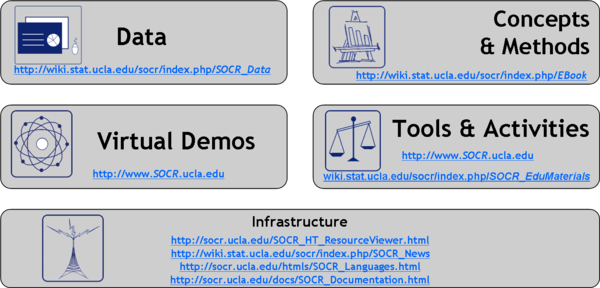# SOCR Events July2008

## Objectives

The specific aims of this hands-on presentation are to discuss the use of the free and integrated Internet-based SOCR Resources for## Use basic concepts of probability to determine the likelihood of an event and compare the results of various experiments

• Write the results of a probability experiment as a fraction, ratio, or decimal, between zero and one, or as a percent between zero and one hundred, inclusive
• Compare experimental results with theoretical probability
• Compare individual, small group, and large group results of a probability experiment

## Display and compare data to make predictions and formulate conclusions

• Display data using tables, scatter plots, and circle graphs
• Compare two similar sets of data on the same graph
• Compare two different kinds of graphs representing the same set of data
• Propose and justify inferences and predictions based on data

## Calculate probabilities of events and compare theoretical and experimental probability

Use of the Fundamental Counting Principle, complement, theoretical probability, experiment, data, percentile, histogram, box-and-whisker plot, spread

• Solve counting problems using the Fundamental Counting Principle
• Calculate the probability of an event or sequence of events with and without replacement using models
• Recognize that the sum of the probability of an event and the probability of its complement is equal to one
• Make approximate predictions using theoretical probability and proportions
• Collect and interpret data to show that as the number of trials increases, experimental probability approaches the theoretical probability

## Formulate questions and answer the questions by organizing and analyzing data

• Formulate questions that can be answered through data collection and analysis
• Determine the 25th and 75th percentiles (first and third quartiles) to obtain information about the spread of data
• Graphically summarize data of a single variable using histograms and box-and-whisker plots
• Compute the mean and median of a numerical characteristic and relate these values to the histogram of the data
• Use graphical representations and numerical summaries to answer questions and interpret data

## Summarize, display, and analyze bivariate data

Use of scatter plot, positive correlation, negative correlation, no correlation, line of best fit, bivariate data.

• Collect, record, organize, and display a set of data with at least two variables
• Determine whether the relationship between two variables is approximately linear or nonlinear by examination of a scatter plot
• Characterize the relationship between two linear related variables as having positive, negative, or approximately zero correlation
• Estimate, interpret, and use lines fit to bivariate data
• Estimate the equation of a line of best fit to make and test conjectures
• Interpret the slope and y-intercept of a line through data
• Predict y-values for given x-values when appropriate using a line fitted to bivariate numerical data

## Apply basic concepts of probability

Use of permutation, combination, conditional probability, discrete random variable, standard deviation, interquartile range, percentile.

• Distinguish between permutations and combinations and identify situations in which each is appropriate
• Calculate probabilities using permutations and combinations to count events
• Compute conditional and unconditional probabilities in various ways, including by definitions, the general multiplication rule, and probability trees
• Define simple discrete random variables

## Use percentiles and measures of variability to analyze data

• Compute different measures of spread, including the range, standard deviation, and interquartile range
• Compare the effectiveness of different measures of spread, including the range, standard deviation, and interquartile range in specific situations
• Use percentiles to summarize the distribution of a numerical variable
• Use histograms to obtain percentiles

## Compute probabilities for discrete distributions and use sampling distributions to calculate approximate probabilities

• Obtain sample spaces and probability distributions for simple discrete random variables.
• Compute binomial probabilities using Pascal’s Triangle and the Binomial Theorem.
• Compute means and variances of discrete random variables.
• Compute probabilities using areas under the Normal Curve.
• Calculate parameters of sampling distributions for the sample average, sum, and proportion.
• Calculate probabilities in real problems using sampling distributions.

## Analyze bivariate data using linear regression methods

• Fit regression lines to pairs of numeric variables and calculate the means and standard deviations of the two variables and the correlation coefficient, using technology.
• Compute predictions of y-values for given x-values using a regression equation, and recognize the limitations of such predictions.